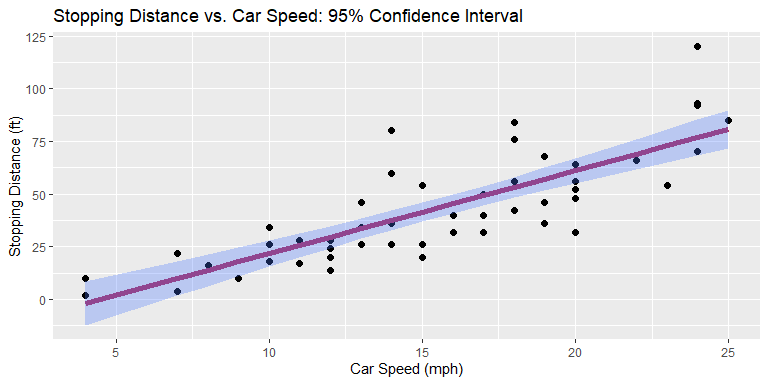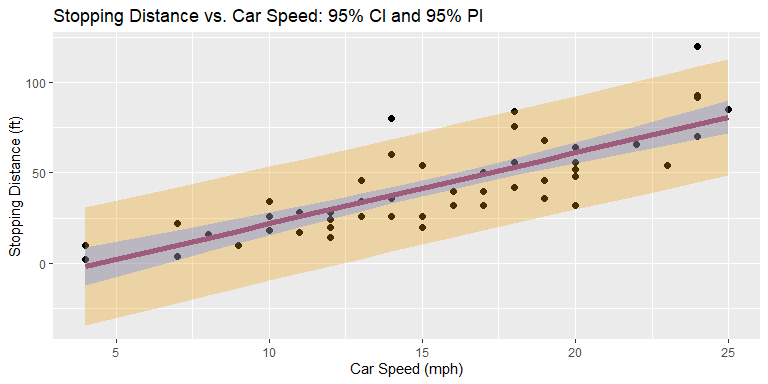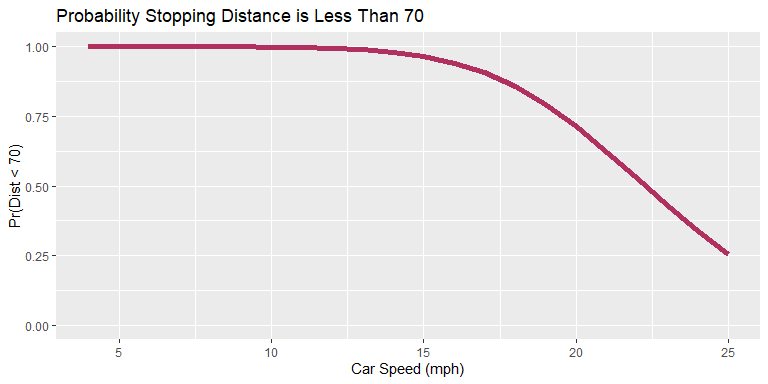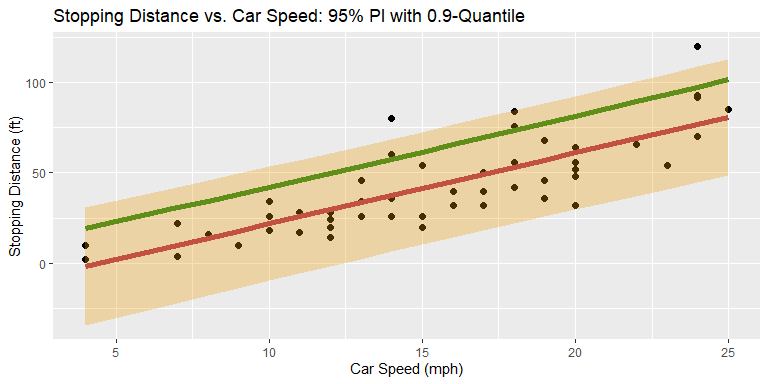# Overview

The purpose of ciTools is to make it easier to do common types of inference in R, particularly uncertainty bounds and probability and quantile estimates. These are the tools researchers use when comparing average system performance to requirements, bounding future system performance, and estimating whether the system will achieve specific thresholds that aren’t necessarily average performance. Specifically, ciTools gives users access to one-line commands that produce confidence intervals and prediction bounds for a given design matrix. This matrix can be the observed data from a test or a set of points that “span the space”, allowing the analyst to visualize system performance. For more information about spanning a design space in R, see data_grid or crossing.

ciTools makes these statistical quantities available through a set of four functions that have a uniform syntax: add_<*>(data, model, ...). Users only need to learn one expression to get started and can intuitively learn other functions when needed.

# Using ciTools

We designed ciTools to make it easy and convenient to generate intervals estimates when you’re done building a model. Since the exact formulation of a confidence interval depends on the type of statistical model fit, figuring out how to construct a proper confidence interval can be challenging. These functions automatically identify the correct interval for you, provided your model is one of the supported classes.

Here are the four main functions of ciTools that you can use regardless of the type of model you made:

1. add_ci(data, model, ...) – compute confidence intervals for the fitted values of each row in data and append to data.
2. add_pi(data, model, ...) – compute prediction intervals for the fitted values of each row in data and append to data.
3. add_probs(data, model, ...) – compute conditional response probabilities for the fitted values of each row in data and append to data.
4. add_quantiles(data, model, ...) – compute conditional response quantiles for the fitted values of each row in data and append to data.

In each of the above functions, model is the model you’ve fit (of class lm, glm, or lmerMod, which correspond to models fit with the functions lm, glm, and lmer respectively), and data is the matrix of data points for which you’d like uncertainty estimates.

Each function returns data with your estimates appended to facilitate plotting with ggplot. For those familiar with the modelr package, they function the same way as add_predictions. Another advantage is to make all of these commands interoperable: they may be chained together to give all the quantities of interest at once.

# Example

Here we will feature a common linear model in R that uses the cars dataset. Uncertainty intervals in R for linear models are well supported through the functions predict.lm, so the functions we provide in ciTools are “wrappers” over predict.lm.

my_data <- cars
glimpse(my_data)
## Rows: 50
## Columns: 2
## $speed <dbl> 4, 4, 7, 7, 8, 9, 10, 10, 10, 11, 11, 12, 12, 12, 12, 13, 13,... ##$ dist  <dbl> 2, 10, 4, 22, 16, 10, 18, 26, 34, 17, 28, 14, 20, 24, 28, 26,...

A linear model that estimates stopping distance as a function of speed:

model <- lm(dist ~ speed, data = cars)

## Confidence Intervals

If we were interested in the average stopping distance as a function of speed, we can generate a confidence interval using add_ci(). The output is another data frame with three new columns: one for the model predictions, one for the lower confidence bound, and one for the upper confidence bound:

my_data_with_ci <- add_ci(my_data, model, names = c("lcb", "ucb"))
kable(head(my_data_with_ci, n =10), row.names = TRUE)
speed dist pred lcb ucb
1 4 2 -1.849460 -12.329543 8.630624
2 4 10 -1.849460 -12.329543 8.630624
3 7 4 9.947766 1.678977 18.216556
4 7 22 9.947766 1.678977 18.216556
5 8 16 13.880175 6.307527 21.452823
6 9 10 17.812584 10.905121 24.720047
7 10 18 21.744993 15.461917 28.028068
8 10 26 21.744993 15.461917 28.028068
9 10 34 21.744993 15.461917 28.028068
10 11 17 25.677401 19.964525 31.390278

The data and the model fit can be inspected graphically with ggplot:

my_data_with_ci %>%
ggplot(aes(x = speed, y = dist)) +
geom_point(size = 2) +
geom_line(aes(y = pred), size = 2, color = "maroon") +
geom_ribbon(aes(ymin = lcb, ymax = ucb), fill =
"royalblue1", alpha = 0.3) +
ggtitle("Stopping Distance vs. Car Speed: 95% Confidence Interval") +
xlab("Car Speed (mph)") +
ylab("Stopping Distance (ft)")The red line is the model’s estimated average stopping distance across the different car speeds, and the blue region represents the uncertainty in the average stopping distance. The default confidence level is 95 percent. Users who desire a different confidence level can use the option alpha = * to specify a custom level. For example, if 80 percent intervals are desired, then include alpha = 0.2 in the add_ci() call.

## Prediction Intervals

Prediction intervals are similar to confidence intervals, but instead of conveying the uncertainty in the estimated average, prediction intervals convey the uncertainty in a new observation. There is more uncertainty about a single new observation than the average of all new observations, so prediction intervals are wider than confidence intervals. To generate prediction intervals, use add_pi:

my_data_with_pi <- add_pi(my_data, model, names = c("lpb", "upb"))

The data frame is now larger because we have two new columns for lower and upper prediction bounds tacked on to the end:

kable(head(my_data_with_pi, n = 10), row.names = TRUE)
speed dist pred lpb upb
1 4 2 -1.849460 -34.499842 30.80092
2 4 10 -1.849460 -34.499842 30.80092
3 7 4 9.947766 -22.061423 41.95696
4 7 22 9.947766 -22.061423 41.95696
5 8 16 13.880175 -17.956287 45.71664
6 9 10 17.812584 -13.872245 49.49741
7 10 18 21.744993 -9.809601 53.29959
8 10 26 21.744993 -9.809601 53.29959
9 10 34 21.744993 -9.809601 53.29959
10 11 17 25.677401 -5.768620 57.12342

Here is what it looks like when we represent confidence intervals and prediction intervals at the same time:

my_data %>%
add_ci(model, names = c("lcb", "ucb")) %>%
add_pi(model, names = c("lpb", "upb")) %>%
ggplot(aes(x = speed, y = dist)) +
geom_point(size = 2) +
geom_line(aes(y = pred), size = 2, color = "maroon") +
geom_ribbon(aes(ymin = lpb, ymax = upb), fill = "orange2",
alpha = 0.3) +
geom_ribbon(aes(ymin = lcb, ymax = ucb), fill =
"royalblue1", alpha = 0.3) +
ggtitle("Stopping Distance vs. Car Speed: 95% CI and 95% PI") +
xlab("Car Speed (mph)") +
ylab("Stopping Distance (ft)")In the graph above, the blue confidence intervals show the uncertainty in the model fit itself (maroon line), and the orange prediction intervals shows where the model would predict 95% of new responses (Stopping Distances) to fall.

## Response Probabilities

Often we want other quantities that depend on the conditional predictive distribution. These include response-level probabilities and response-level quantiles, which are accessed with the functions add_probs and add_quantile respectively.

For example, suppose in the cars data set, I want to know: For each Speed what is the probability that a new Stopping Distance will be less than 70 feet? This may be an important question if, for example, you’re in a car that is hurdling towards a cliff 70 feet away at some speed and you want to know what the probability is that you will be able to stop the car before going over the cliff. Luckily, with add_probs(), you can generate these estimates quickly, allowing you to understand your chance of survival before the problem becomes moot:

my_data %>%
ggplot(aes(x = speed, y = prob_less_than70)) +
geom_line(aes(y = prob_less_than70), size = 2, color = "maroon") +
scale_y_continuous(limits = c(0,1)) +
ggtitle("Probability Stopping Distance is Less Than 70") +
xlab("Car Speed (mph)") +
ylab("Pr(Dist < 70)")The new argument q = * is used to specify the quantile (70 feet in this case) used for computing the probabilities. It’s clear that the probability of surviving declines quickly after 15 mph. (This data set is from the 1920s. It is safer to drive toward cliffs in today’s vehicles).

Another optional argument is comparison, which defaults to "<". In this example, we wanted to know the probability that we’d be able to stop the car before it went over the cliff, which means a stopping distance less than 70 feet. If we were to specify comparison = ">", then add_probs() would return the probability that the stopping distance was greater than 70 feet.

## Response Quantiles

On the other hand, suppose my car is hurdling toward a cliff, and I’m comfortable with stopping at whatever distance guarantees about 90% survivability given my speed. These distances are called response quantiles, and what we wish to compute is the 0.9-quantile (or the 90th percentile) of the distibution of Stopping Distances conditional on my car’s speed and the linear model. In ciTools we use the function add_quantile with the argument p = 0.9 to calculate the 90th percentile of the predictive distibution for each row in the data set. These quantiles will be parallel to the bounds of the prediction intervals that we calculated previously.

my_data %>%
add_pi(model, names = c("lpb", "upb")) %>%
ggplot(aes(x = speed, y = dist)) +
geom_point(size = 2) +
geom_line(aes(y = pred), size = 2, color = "maroon") +
geom_line(aes(y = quantile0.9), size = 2, color = "forestgreen") +
geom_ribbon(aes(ymin = lpb, ymax = upb), fill = "orange2",
alpha = 0.3) +
ggtitle("Stopping Distance vs. Car Speed: 95% PI with 0.9-Quantile") +
xlab("Car Speed (mph)") +
ylab("Stopping Distance (ft)")The 0.9 quantile lies slightly below the upper prediction bound, which in this case is the same as the 0.975-quantile. The red line is the prediction the linear model makes, which is the same as the 0.5-quantile in this case because our model assumes normally distributed errors.

The examples above have taken each piece of analysis one step at a time. However, because ciTools was built to be fully compatible with the tidyverse, these functions can easily be chained or “piped” together in clear, legible code:

data_with_results <- my_data %>%

kable(head(data_with_results))
speed dist pred LCB0.025 UCB0.975 LPB0.025 UPB0.975 prob_less_than70 quantile0.9
4 2 -1.849460 -12.329543 8.630624 -34.49984 30.80092 0.9999723 19.25192
4 10 -1.849460 -12.329543 8.630624 -34.49984 30.80092 0.9999723 19.25192
7 4 9.947766 1.678977 18.216556 -22.06142 41.95696 0.9997778 30.63476
7 22 9.947766 1.678977 18.216556 -22.06142 41.95696 0.9997778 30.63476
8 16 13.880175 6.307527 21.452823 -17.95629 45.71664 0.9995553 34.45554
9 10 17.812584 10.905121 24.720047 -13.87224 49.49741 0.9991164 38.28995

# Scope of ciTools

ciTools handles more than just linear models but the workflow is the same as the example above. Here is current status of development:

Models Confidence Intervals Prediction Intervals Response Probabilities Response Quantiles
Linear [X] [X] [X] [X]
Log-Linear [X] [X] [X] [X]
GLM [X] [X] [X] [X]
Linear Mixed Model [X] [X] [X] [X]
Log-Linear Mixed [TODO] [X] [X] [X]
Survival [X] [X] [X] [X]
Generalized Linear Mixed [X] [X] [X] [X]
• [X] = complete, available now

# Installation

Open up R and run:

1. install.packages("ciTools")
2. library(ciTools)